# CBSE Class 9 Science Physical Science Notes

Download CBSE Class 9 Science Physical Science Notes in PDF format. All Revision notes for Class 9 Science have been designed as per the latest syllabus and updated chapters given in your textbook for Science in Standard 9. Our teachers have designed these concept notes for the benefit of Grade 9 students. You should use these chapter wise notes for revision on daily basis. These study notes can also be used for learning each chapter and its important and difficult topics or revision just before your exams to help you get better scores in upcoming examinations, You can also use Printable notes for Class 9 Science for faster revision of difficult topics and get higher rank. After reading these notes also refer to MCQ questions for Class 9 Science given our website

PHYSICAL SCIENCE

This science deals with the properties and Behaviour of nonliving things.

(a) Physics (in Greek Nature): It is the branch of science which deals with the study of the natural laws and their manifestation in the natural phenomenon.

Mechanics (oldest branch): If deals with the conditions of rest or motion of the material objects around us.

Statics: It deals with the study of object at rest or in equilibrium, even when they are under the action of several forces (measurement of time is not essential).

Kinematics: If deals with the study of motion of objects without considering the cause of motion measurement of time is essential).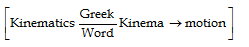Dynamics: It deal with the study of objects taking into consideration the cause of their motion.Rest: An object is said to be at rest if it does not change its position w.r.t. its surroundings with the passage of time.

Motion: A body is said to be in motion if its position changes continuously w.r.t. the surroundings (or with respect to an observer) with the passage of time.

REST AND MOTION ARE RELATIVE TERMS

Eg. : 1 A, B and C are three persons. B and C are sitting in the car and A is standing outside it. When car starts to move, B and C are changing their position with respect to A so B and C are in motion with respect to A but B is not changing its position with time with respect to C, so B is at rest with respect to C (same for C). Therefore motion depends on the position of the observer , hence motion is relative.

Eg. :2 We know that the earth is rotating about its axis and revolving around the sun. The stationary objects like your classroom, a tree and the lamp posts etc., do not change their position with respect to each other i.e. they are at rest. Although earth is in motion. To an observer situated outside the earth, say in a space ship, our classroom, trees etc. would appear to be in motion. Therefore, all motions are relative. There is nothing like absolute motion.

(a) Concept of a Point Object :

In mechanics while studying the motion of an object, sometimes it dimension are of no importance and the object may be treated as point object without much error. When the size of the object is much less in comparison to the distance covered by the object then the object is considered as a point object.

Eg. : 1 If one travels by a car from one place to another far away place, then length of the car is ignored as compared to distance traveled.

Eg. : 2 Earth can be regarded as a point object for studying its motion around the sun.

(b) Frame of Reference :

To locate the position of object we need a frame of reference. A convenient way to set up a frame of reference is to choose three mutually perpendicular axis and name them x-y-z axis. The coordinates (x, y, z) of the particle then specify the position of object w.r.t. that frame. If any one o more coordinates change with time, then we say that the object is moving w.r.t. this frame.

MOTIONS IN ONE, TWO AND THREE DIMENSIONS (TYPE OF MOTION)

As position of the object may change with time due to change in one or two or all the three coordinates, so we have classified motion as follows :

(a) Motion in 1-D:

If only one of the three co-ordinates specifying the position of object changes w.r.t. time. In such a case the object moves along a straight line and the motion therefore is also known as rectilinear or linear motion.

Eg. :  (i) Motion of train along straight railway track.

(ii) An object falling freely under gravity.

(iii) When a particle moves from P1 to P2 along a straight line path only the x-co-ordinate changes(b) Motion in 2-D:

If two of the three co-ordinates specifying the position of object changes w.r.t. time, then the motion of object is called two dimensional. In such a motion the object moves in a plane.

Eg. : (i) Motion of queen on carom board.

(ii) An insect crawling on the floor of the room.

(iii) Motion of object in horizontal and vertical circles etc.

(iv) Motion of planets around the sun.

(v) A car moving along a zigzag path on a level road.

(c) Motion is 3-D:

If all the three co-ordinates specifying the position of object changes w.r.t. time, then the motion of object is called 3-D. In such a motion the object moves in a space.

Eg.: (i) A bird flying in the sky (also kite).

(ii) Random motion of gas molecules.

(iii) Motion of an aeroplane in space.

TYPES OF MOTION

(i) Linear motion (or translatory motion) : The motion of a moving car, a person running, a stone being dropped.

(ii) Rotational motion : The motion of an electric fan, motion of earth about its own axis.

(iii) Oscillatory motion : The motion of a simple pendulum, a body suspended from a spring (also called to and fro motion).

SCALAR AND VECTOR QUNTITY

Physically quantities (i.e. quantities of physics) can be divided into two types :

(i) Scalar quantity : Any physical quantity, which can be completely specified by its magnitude alone, is a scalar quantity or a scalar.

Eg.:Charge, distance, area, speed, time temperature, density, volume, work, power, energy, pressure, potential etc.

(ii) Vector quantity : Any physical quantity, which requires direction in addition to its magnitude is known as a vector.

Eg. :Displacement, velocity, acceleration, force, momentum, weight and electric field etc.

(a) Representation of a vector :

A vector is represented a directed line segment drawn in the given direction on a certain scale.

Eg.: To represent a displacement of 50 m towards east. Take 10 m = 1 cm (Scale)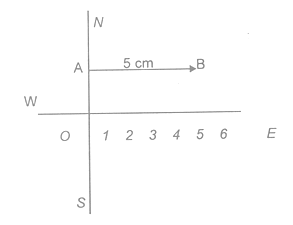Eg.:  To represent a velocity of 20 k m/h towards 300 east of south. (Scale 5 km/h = 1 cm.)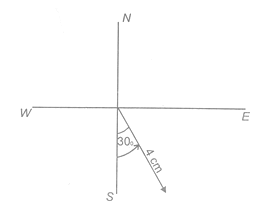Eg. : 6 m displacement, 600 north - east (north of east) (Scale 1 m = 1 cm)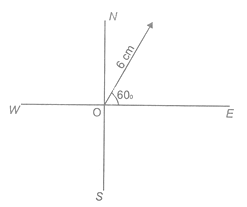(b) Difference between Scalar and Vector :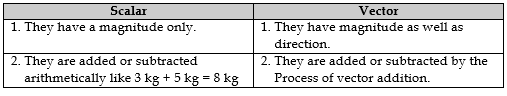DISTANCE AND DISPLACEMENT

(a) Distance :

Consider a body traveling from A to B along any path between A & B. The actual length of the path that a body travels between A and B is known as the distance. The distance traveled is different for different path between A and B. It is a scalar quantity. According to figure distance at path APB is AP + PB and at path AB is AB(b) Displacement :

The distance traveled in a given direction is the displacement. Thus displacement is the shortest distance between the given points. It is a vector quantity. S.I. unit of distance or displacement is metre.

NOTE : If a body travels in such a way that it comes back to its starting position, then the displacement is zero. However, distance traveled is never zero.

Eg. :(i) When an object moves towards right from origin to in time t1 to t2, its displacement is positive.(ii) When an object moves towards left in time t1 to t2, its displacement is negative.(iii) When an object remains stationary or it moves first towards right and then an equal distance towards left, its displacement is zero.

(iv) Shifting origin causes no change in displacement.

(c) Difference between Distance and Displacement :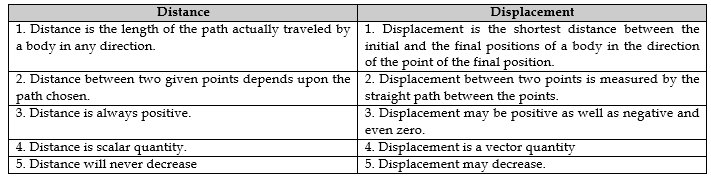UNIFORM AND NON UNOFORM MOTION

(a) Uniform Motion :

A body has a uniform motion if it travels equal distances in equal intervals of time, no matter how small these time intervals may be. For example, a car running at a constant speed of say, 10 meters per second, will cover equal distances of 10 metres every second, so its motion will be uniform. Please note that the distance-time graph for uniform motion is a straight line (as shown in the figure).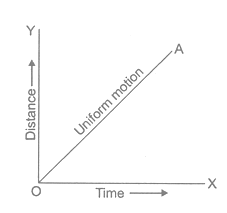(b) Non-Uniform Motion :

body has a non-uniform if it travels unequal distances in equal intervals of time. For example, if we drop a ball from the roof of a building, we will find that it covers unequal distances in equal intervals of time. It covers :

4.9 metres in the 1st second,

14.7 metres in the 2nd second,

24.5 metres in the 3rd second, and so on.

Thus, a freely falling ball covers smaller distance in the initial ‘1 second’ interval and larger distance in the later ‘1 second’ interval. From this discussion we conclude that the motion of a freely falling body is an example of non-uniform motion. The motions of a train starting from the railway station is also an example of non-uniform motion. This is because when the train starts from a s station, if moves a very small distance in the ‘first’ second. The train moves a little more distance in the ‘2nd’ second and so on. And when the train approaches the next station, the distance traveled by it per second decreases.Please note that the distance-time graph for a body having non-uniform motion is curved line (as shown in the figure). Thus, in order to find out whether a body has uniform motion or non-uniform motion, we should draw the distance-time graph for it. If the distance time graph is straight line, the motion will be uniform and if the distance -time graph is a curved line, the motion will be non-uniform. It should be noted that non-uniform motion is also called accelerated motion.

SPEED

The distance traveled by a body in unit time is called its peed. Therefore,

speed = Distance/ Time   or s = d/t. S.I. unit of speed or average speed is m/sec. It is a scalar quantity,

(a) Average Speed :

For an object moving with variable speed, it is the total distance traveled by the object divided by the total time taken to cover that distance.

Average speed = total distance  travelled / total  time taken

(b) Uniform Speed (or Constant Speed):

When an object covers equal distance in equal intervals of time, it is said to move with uniform speed.

Eg. A car moves 10 m is every one second so it motion is uniform.

(c) Variable Speed (Non-Uniform Speed) :

If a body covers unequal distance in equal intervals of time, its motion is said to be non-uniform.

Eg.Falling of a apple from a tree, a cyclist moving on a rough road, an athlete running a race, vehicle starting from rest, the motion of freely falling body etc.

(d) Instantaneous Speed :

The speed of an object at any particular instant of time or at particular point of its path is called the instantaneous speed of the object. it is measure red by speedometer in an automobile.

VELOCITY

It is the rate of change of displacement.

Therefore, velocity =displacement/ time  or it is the distance traveled in unit time in a given direction.

velocity = distance travelled in a given direction / time taken

S.I. unit of velocity is  m/s. If is a vector quantity.

(Magnitude of the velocity is known as speed) 1 km/h = 5/18 m/s.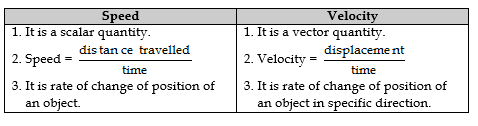(a) Uniform Velocity (Constant Velocity) :

If a body covers equal distance in equal intervals of time in a given direction then it is said to be moving with constant velocity.

(b) Non-Uniform Velocity :

When a body does not cover not cover equal distances in equal intervals of time, in a given direction (in this case speed is not constant), then it is known as non  uniform velocity. If speed is constant then also body can have a non-uniform velocity.

Eg : A car moving on a circular road with constant speed.

(c) Average Velocity :

If initial velocity of body is u and final velocity is v then the arithmetic means of velocity is called average velocity and is given as.v2v = u+v/2 Where, u = initial velocity and v = final velocity. Also for an object moving with variable velocity it is defined as the ratio of its total displacement to the total time interval in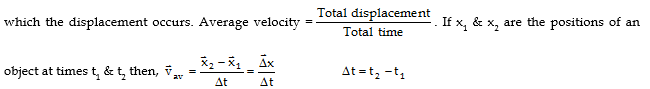(d) Instantaneous Velocity :

The velocity of an object at any given instant of time at particular point of its path is called its instantaneous velocity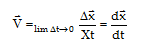Ex.When is the average speed of an object equal to the magnitude of its average velocity ? Give reason also.

Sol.  As average speed =  total pathlength /  time interval also, average velocity = Displacement/ time interval . When an object moves along a straight line and in the same direction its total path length is equal to the magnitude of its displacement. Hence average speed is equal to the magnitude of its average velocity.

FEATURE OF UNIFORM MOTION

(i) The velocity in uniform motion does not depend on the choice of origin.

(ii) The velocity in uniform motion does not depend on the choice of the time interval (t2 – t1).

(iii) For uniform motion along a straight line in the same direction, the magnitude of the displacement is equal to the actual distance covered by the object.

(iv) The velocity is positive if the object is moving towards the right of the origin and negative if the object is moving towards the left of the origin.

(v) For an object is uniform motion no force is required to maintain its motion.

(vi) In uniform motion, the instantaneous velocity is equal to the average velocity at all time because velocity remains constant at each instant, at each point of the path.

ILLUSTRATIONS

1. A car is moving along x-axis. As shown in figure it moves from O to P in 18 s and returns from P to Q in 6 second. What is the average velocity and average speed of the car in going from (i) O to P and (ii) from O to P and back to Q.2.A car covers the 1st half of the distance between two places at a speed of 40 km h-1 and the 2nd half at 60 km h-1. What is the average speed of the car ?

Sol. Suppose the total distance covered is 2S.

Then time taken to cover first distance with speed 40 km/h,

t1= S/ 40 h

Time taken to cover second S distance with speed 60 km/h,3.A non-stop bus goes from one station to another station with a speed of 54 km/h, the same bus returns from the second station to the first station with a speed of 36 km/h. Find the average speed of the bus for the entire journey.

Sol.Suppose the distance between the stations is S. Time taken in reaching from one station to another station.ACCELERATION

Mostly the velocity of a moving object changes either in magnitude or in direction or in both when the object moves. The body is then said to have acceleration. So it is the rate of change of velocity i.e. change in velocity in unit time to the acceleration (it is a vector quantity). Its S.I. unit is m/sec2 and c.g.s unit is c m/sec.2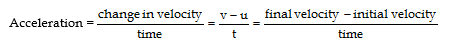(a) Uniform Acceleration (Uniformly Accelerated Motion):

If a body travels in a straight line and its velocity increases in equal amounts in equal intervals of time. Its motion is known as uniformly accelerated motion.

Eg.1  Motion of a freely falling body is an example of uniformly accelerated motion (or motion of a body under the gravitational pull of the earth).

Eg.2  Motion of a bicycle going down the slope of a road when the rider is not pedaling and wind resistance is negligible.

(b) None-Uniform Acceleration :

If during motion of a body its velocity increases by unequal amounts in equal intervals of time, then its motion is known as non uniform accelerated motion.

Eg.1Car moving in a crowded street.

Eg.2 Motion of a train leaving or entering the platform.

TYPES OF ACCELERATION

(i) Positive acceleration : If the velocity of an object increases in the same direction, the object has a positive acceleration.

(ii) Negative acceleration (retardation): If the velocity of a body decreases in the same direction, the body has negative acceleration or it is said to be retarding.

Eg.A train slows down.

EQUATIONS OF UNIFORMLY ACCELERATION MOTION

(a) 1st Equation of Motion :

Consider a body having initial velocity ‘u’. Suppose it is subjected to a uniform acceleration ‘a’ so that after time ‘t’ its final velocity becomes ‘v’. Now we now,

Acceleration = change in  velocity / time

a= v-u / t

or     v = u + at  or  v = at + u           …..(i)

(b) 2nd Equation of Motion :

Suppose a body has an initial velocity ‘u’ and uniform acceleration ‘a’ for time ‘t’ so that its final velocity becomes ‘v’. The distance traveled by moving body in time ‘t’ is ‘s’ then the average velocity = (v + u)/2. Distance traveled = Average velocity × time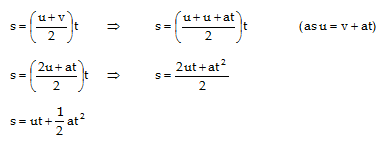(c) 3rd Equation of Motion

Distance travelled = Average velocity × timed) Distance  covered  in nth second :

S= ut+ 1/2  at2 is the distance  covered by a body n t s.TO SOLVE NUMERIAL PROBLEMS

(i)If a body is dropped from a height then its initial velocity u = 0 but has acceleration (acting). If a body starts from rest its initial velocity u = 0.

(ii)   If a body comes to rest, its final velocity v = 0 or, if a body reached the highest point after being thrown upwards its final velocity v = 0 but has acceleration (acting).

(iii) if a body moves with uniform velocity, its acceleration is zero i.e. a = 0.

(iv) Motion of body is called free fall if only force acting on it is gravity (i.e. earth’s attraction).

MOTION UNDER GRAVITY (UNIFORM ACCELERATED MOTION)

The acceleration with which a body travels under gravity is called acceleration due to gravity ‘g’. Its value is 9.8 m/s2 (or  10 m/s2). If you have to take g = 10 m/s2 then it must be mentioned in the question otherwise take g = 9.8  m/s2.

(i) If a body moves upwards (or thrown up) g is taken negative (i.e. motion is against gravitation of earth). So we can form the equation of motion like.

v = u - gt, s = ut - 1/2 gt2, v2 - u= - 2 gh.

(ii) If a body travels downwards (towards earth) then g is taken + ve. So equations of motion becomes v = u + gt, s = ut +1/2  gt2, v2 - u2 = 2gh.

(iii) if a body is projected vertically upwards with certain velocity then it returns to the same point of projection with the same velocity in the opposite direction.

(iv) The time for upward motion is the same as for the downward motion.

ILLUSTRATION

1.A car is moving at a speed of 50 km/h. Two seconds there after it is moving at 60 km/h. Calculate the acceleration of the car.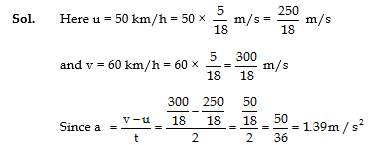2. A car attains 54 km/h in 20 s after it starts. Find the acceleration of the car.3.A ball is thrown vertically upwards with a velocity of 20 m/s. How high did the ball go ? (take g = 9.8 m/s2).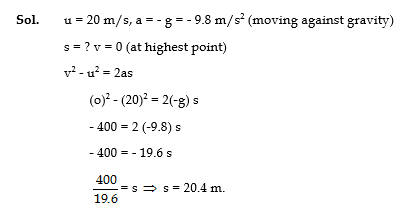DISTANCE (DISPLACEMENT) FROM SPEED (VELOCITY) TIME GRAPH

A distance (displacement = speed (velocity) x time, so the distance (displacement) can be calculated (computed) with speed (velocity) - time graph.

Case (i) : When speed (velocity) is uniform (constant):

Figure shows the speed - time graph of a car (taxi) moving with a uniform speed of 50 km h-1. It is a straight line parallel to X - axis (time axis). Distance covered by this taxi from time t1 = 4h at P to time t2 = 8 h at S, is given by distance = 50 × (t2 - t1)

= 50 (8 - 4)

= 50 × 4 = 200 km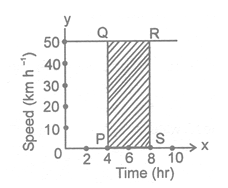In figure, PQ = 50, SP = (t1 - t1)

Hence distance = PQ × SP = Area of rectangle PSRQ

Case (ii) : When speed (velocity) as well as acceleration is non-uniform (variable)

Figure shows the speed- time graph of a body moving with variable speed and acceleration. Over a small interval of time, the speed can be taken as constant. For this small time interval, distance = Area of the blackened strip.For whole time-interval between t1 and t2

distance = sum of area of all the strips between t1 and t2 = Area of shaded figure PQRS.

GRAPHICAL DERIVATION OF EQUATIONS OF MOTION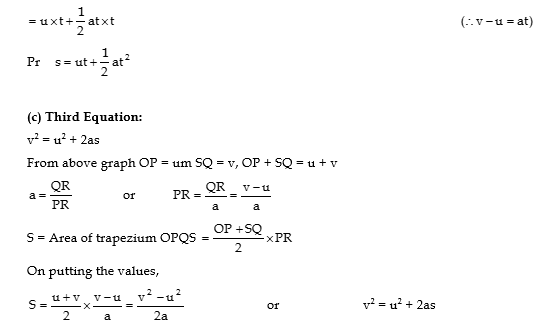CIRCULAR MOTION

(a) Definition :

Motion of a particle (small body) along a circle (circular path), is called a circular motion. If the body covers equal distances along the circumference of the circle in equal intervals of time, the motion is said to be a uniform circular motion. A uniform circular motion is a motion in which speed remains constant but direction of velocity changes.

(b) Explanation :

Consider a boy running along a regular hexagonal track (path) as shown in figure. As the boy runs along the side of the hexagon at a uniform speed, he has to take turn at each corner changing direction but keeping the sped same. In one round he has to take six turns at regular intervals. If the same boy runs along the side of a regular octagonal track with same uniform speed, he will have to take eight turns in one round at regular intervals but the interval will become smaller.By increasing the number of sides of the regular polygon, we find the number of turns per round becomes more and the interval between two turns become still shorter. A circle is a limiting case of polygon with an infinite number of sides. On the circular track, the turning becomes a continuous process without any gap in between. The boy running along the sides of such a track will be performing a circular motion. Hence, circular motion is the motion of a body along the sides of polygon of infinite number of sides with uniform speed, the direction changing continuously.

Eg.Example of uniform circular motion are :

(i) Motion of moon around the earth.

(ii) Motion of satellite around its planet.

(c) Nature of Circular of Motion :

Circular motion is an acceleration motion. Since, in a circular motion, velocity changes though in direction only, the motion is said to be accelerated.

DIFFERENCE BETWEEN UNIFORM LINEAR MOTION AND A UNIFOR CIRCULAR MOTION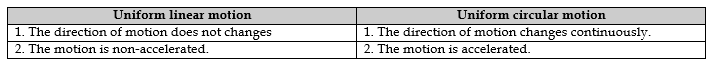RADIAN - (A UNIT FOR PLANE ANGLE)

It is a convenient unit for measuring angle in physics.

(a) Definition:

One radian is defined as the angle subtended at the centre of the circle by an arc equal in length to its radius.

Eg. In figure, the arc AB of the circle has length  and subtends an angle  at the centre C.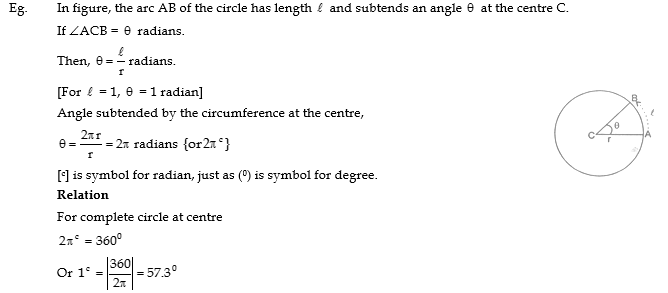ANGULAR DISPLACEMENT AND ANGULAR VELOCITY

(a) Definitions :

(i) Angular displacement : In a circular motion, the angular displacement of a body is the angle subtended by the body at the centre in a given interval of time. It is represented by the symbol  θ(theta).

(ii) Angular velocity : The angular displacement per unit time is called the angular velocity. it is represented by the symbol ω (omega).

Eg. Let a body move along a circle of radius r and perform a uniform circular motion. Let the body be at point P to start with and reach point Q after time t. Then, angular displacement = <PCQ =θ and angular velocity(b) Units for θ and ω :

The unit for angular displacement is radian (a supplementary quantity). The radian is defined at the angle subtended at the centre of a circle by an arc equal in length to its radius. The unit from angular velocity radian per second (rad/s).

(c) Relation between Linear and Angular Quantities: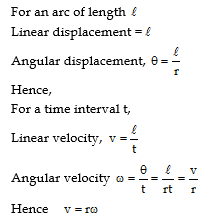## Tags:

Click for more Science Study Material
 CBSE Class 9 Science Atoms And Molecules Notes Set A CBSE Class 9 Science Atoms And Molecules Notes Set B CBSE Class 9 Science Categories Of Practical Skills Notes CBSE Class 9 Science Diversity In Living Organisms Notes Set A CBSE Class 9 Science Diversity In Living Organisms Notes Set B CBSE Class 9 Science Force And Laws Of Motion Notes Set A CBSE Class 9 Science Force And Laws Of Motion Notes Set B CBSE Class 9 Science Fundamental Unit Of Life Notes CBSE Class 9 Science Gravitation And Fluid Notes Set A CBSE Class 9 Science Gravitation And Fluid Notes Set B CBSE Class 9 Science Gravitation And Fluid Notes Set C CBSE Class 9 Science Improvement In Food Resources Notes Set A CBSE Class 9 Science Improvement In Food Resources Notes Set B CBSE Class 9 Science Is Matter Around Us Pure Notes Set A CBSE Class 9 Science Is Matter Around Us Pure Notes Set B CBSE Class 9 Science List Of Experiments CBSE Class 9 Science Matter In Our Surroundings Notes Set A CBSE Class 9 Science Matter In Our Surroundings Notes Set B CBSE Class 9 Science Motion Notes CBSE Class 9 Science Motion Notes Set A CBSE Class 9 Science Natural Resources Notes Set A CBSE Class 9 Science Natural Resources Notes Set B CBSE Class 9 Science Physical Science Notes CBSE Class 9 Science Revision Notes CBSE Class 9 Science Sound Notes CBSE Class 9 Science Structure Of Atom Notes Set A CBSE Class 9 Science Structure Of Atom Notes Set B CBSE Class 9 Science The Fundamental Unit Of Life Notes CBSE Class 9 Science Tissue Notes Set A CBSE Class 9 Science Tissue Notes Set B CBSE Class 9 Science Wave Motion And Sound Notes CBSE Class 9 Science Why Do We Fall Ill Notes Set A CBSE Class 9 Science Why Do We Fall Ill Notes Set B CBSE Class 9 Science Work Energy And Power Notes Set A CBSE Class 9 Science Work Energy And Power Notes Set B

## Latest NCERT & CBSE News

Read the latest news and announcements from NCERT and CBSE below. Important updates relating to your studies which will help you to keep yourself updated with latest happenings in school level education. Keep yourself updated with all latest news and also read articles from teachers which will help you to improve your studies, increase motivation level and promote faster learning

### Moderation of Marks Class 11 and 12 Board Exams

The portal for moderation and finalization of results for Class-12 is being opened from 16.07.2021 to 22.07.2021. As Board has to declare the result latest by 31.07.2021, schools have been requested to follow the schedule strictly and complete the moderation within...

This has a reference to the letter no CBSE/COORD/2021 dated 11.02.2021 regarding conduct of the School-Based Assessment (Internal Assessment/Practical/ Project) of Class-XII and uploading the marks by 11.06.2021. It has been observed that some schools have not been...

### CBSE Board Examination Scheme of Assessment 2021 2022

A. Academic session to be divided into 2 Terms with approximately 50% syllabus in each term: The syllabus for the Academic session 2021-22 will be divided into 2 terms by following a systematic approach by looking into the interconnectivity of concepts and topics by...

### FAQs on Policy for Tabulation of Marks for Class 10 Board Exams

ON POLICY Question.1 How CBSE will declare the result of Class-X? Answer. Results of Class X Board will be declared on the basis of an objective criterion developed by the Board vide Notification no.CBSE/CE/2021 dated 01.05.2021. Question.2. If any candidate is not...

### CBSE Class 12 Evaluation Criteria 2021

SUMMARY OF THE POLICY FOR TABULATION OF MARKS FOR CLASS XII BOARD EXAMINATIONS 2021   1(a) Due to cancellation of the Board examinations, the assessment of theory portion of 80/70/60/50/30 marks will be done by the school based on the following:     Class...

×